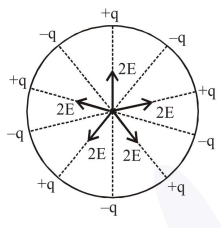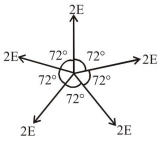# Ten charges are placed on the circumference

Question:

Ten charges are placed on the circumference of a circle of radius $R$ with constant angular separation between successive charges.

Alternate charges $1,3,5,7,9$ have charge $(+\mathrm{q})$ each, while $2,4,6,8,10$ have charge $(-q)$ each. The potential $\mathrm{V}$ and the electric field $\mathrm{E}$ at the centre of the circle are respectively:

(Take $\mathrm{V}=0$ at infinity)

1. $\mathrm{V}=\frac{10 \mathrm{q}}{4 \pi \in_{0} \mathrm{R}} ; \mathrm{E}=\frac{10 \mathrm{q}}{4 \pi \in_{0} \mathrm{R}^{2}}$

2. $\mathrm{V}=0, \mathrm{E}=\frac{10 \mathrm{q}}{4 \pi \epsilon_{0} \mathrm{R}^{2}}$

3. $\mathrm{V}=0, \mathrm{E}=0$

4. $V=\frac{10 q}{4 \pi \epsilon_{0} R} ; E=0$

Correct Option: , 3

Solution:

Potential of centre $=\mathrm{V}=$

$\Sigma\left(\frac{\mathrm{kq}}{\mathrm{R}}\right)$

$\mathrm{V}_{\mathrm{C}}=\frac{\mathrm{K}(\Sigma \mathrm{q})}{\mathrm{R}}$$\mathrm{V}_{\mathrm{C}}=\frac{\mathrm{K}(0)}{\mathrm{R}}=0$

Electric field at centre $\overrightarrow{\mathrm{E}}_{\mathrm{B}}=\Sigma \overrightarrow{\mathrm{E}}$

Let $\mathrm{E}$ be electric field produced by each charge at the centre, then resultant electric field will be$\mathrm{E}_{\mathrm{C}}=0$, Since equal electric field vectors are acting at equal angle so their resultant is equal to zero.